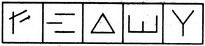# Non Verbal Reasoning - Classification - Discussion

### Discussion :: Classification - Section 1 (Q.No.19)

In each problem, out of the five figures marked (1), (2), (3), (4) and (5), four are similar in a certain manner. However, one figure is not like the other four. Choose the figure which is different from the rest.

19.

Choose the figure which is different from the rest.(1)     (2)     (3)     (4)     (5)

 [A]. 1 [B]. 2 [C]. 3 [D]. 4 [E]. 5

Explanation:

Figure (4) is formed by four line segments while each one of the other figures is formed by three line segments.

 Hamza said: (Mar 26, 2011) The triangle is a closed shape while the rest are not.

 Charan said: (Sep 15, 2011) I think answer is 3. Because triangle forms closed path where as others are not.

 Tod said: (Apr 11, 2014) I picked 3 because the triangle was closed where the other figures were not. The answer is obviously D. These should have more than one correct answer based on perception or more limited options.

 Anmol said: (Jul 26, 2017) Why not option A? because In this two lines intersect each other.

 Preethi said: (Oct 27, 2018) The answer can be (2) because in all the figures expect the (2) figure consist of lines which intersect.

 Ajay Chhetry said: (Mar 13, 2019) Answer 4 is absolutely right because three straight lines are in all except 4.

 Ohm Nabhya said: (Oct 20, 2019) Actually answer is both (c) and (d) because only option (c) is a closed figure but even option (d) is right because it is constructed with 4 line segments while the rest are constructed with 3 line segments.

 Dahil said: (Nov 4, 2020) (c) is the right option which contains only closed figure.

 Surya said: (Jan 2, 2021) Answer can be 3 also because, all are open figures where as traingle is closed one.